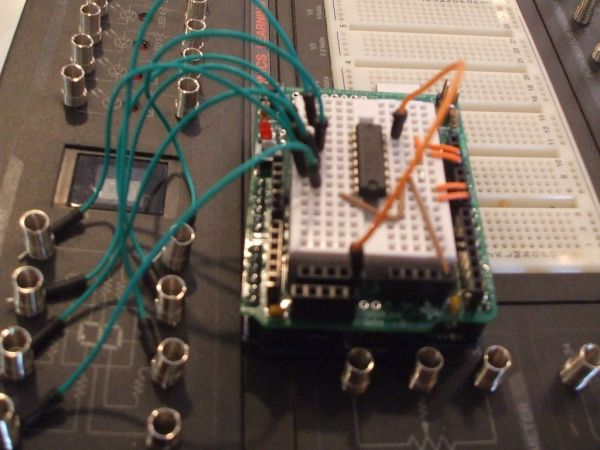# Arduino: an easier way to work with seven segment displays

Ever have trouble programming code for seven segment displays? Well they made the 4511 to make things a whole lot easier. What the 4511 does is takes a 4 digit binary input value (ones and zeros), and converts it to a decimal value on a seven segment display, it’s a really handy chip. In this instructable I’ll show you how to use them with the arduino.## Step 1: Materials

Parts:

1.) Arduino

2.) 4511 binary seven segment decoder

5.) 470 ohm resistor

6.) A to B programming cable

7.) CDS photocell

8.) 10K resistor

Tools:

1.) Computer

2.) A brain 🙂

## Step 2: How the 4511 works

The 4511 takes a four-bit binary input on pins 1,2,6, and 7. Then translates it to a decimal output when connected to a seven segment display, which means that instead of programming 7 individual pins each time the display changes digits, you only have to program four. Also, instead of finding the exact led’s to turn on for each digit, you just enter a binary value, which leads us to the next step:

## Step 3: A thing about binary

For those of you who already are fluent in binary, move on to the next step.

Binary is used in pretty much all mainstream electronics today. It is based on a four digit lines consisting of ones and zeros (each digit is called a bit, each line is called a byte). The first digit in the sequence is equal to one, the second is equal to two, the third is equal to four, and the fourth is equal to eight. So the byte 1001 would be equal to nine (one plus 8). To make it simpler:Binary:    Decimal:
0000   =   0
0001   =   1
0010   =   2
0011   =   3
0100   =   4
0101   =   5
0110   =   6
0111   =   7
1000   =   8
1001   =   9
and so on… (This is as far as the 4511 will go, since it only has one digit capability, it cannot print 10 or up)

## Step 4: A simple timer

The first thing to do is to build a simple proof of concept circuit, a count up timer, and then go from there, for this, you’ll need to have everything exept the CDS photocell and the 10K resistor.

## Step 5: Insert the chip

Insert the chip into the board, make sure to note which way the chip is facing ( where the little notch is o the chip)

### Major Components in Project

1.) Arduino

2.) 4511 binary seven segment decoder

For more detail: Arduino: an easier way to work with seven segment displays